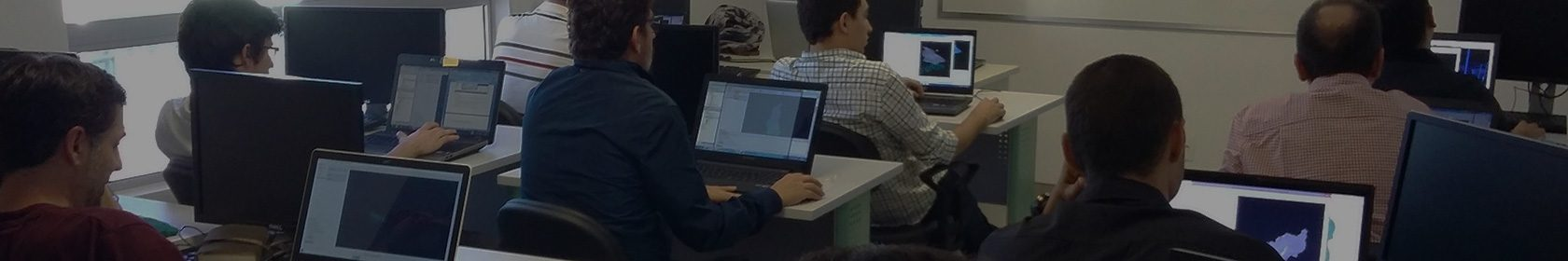# Data Analysis, Mapping and Property Modeling with Geostatistics

Understand and master the use of geostatistics for data analysis and mapping.

## Objectives

At the end of this course, you will be able to use geostatistics to:

• better understand and value your data,
• produce better quality maps by integrating various types of data,
• quantify the uncertainties on the calculated maps,
• understand the underlying assumptions and choose the most suitable geostatistical technique for your data.

## Key Features

• The course is devoted to theoretical and methodological presentations, the second part to practical exercises on real-life cases to deepen the understanding of concepts. The focus is on illustrations and practical contributions of the covered concepts.
• Computer exercises with Isatis.neo software.
• Course material provided (documentation, journal files, training data, worked examples) for re-use in your workplace.

## Who should attend

This course is aimed at those who work with spatial data and wish to understand and put into practice the geostatistical methods for mapping: academics, agricultural engineers, air quality engineers, climatologists, environmental consultants and engineers, epidemiologists, foresters, geotechnical engineers, soil scientists, etc.

## Course content

SESSION 1: ANALYSING DATA AND THEIR VARIABILITY IN SPACE

• Introduction:
– Added-value of geostatistics compared to deterministic methods for spatial interpolation.
– Presentation of usual deterministic interpolation methods (nearest neighbor, moving average, inverse distance, etc.) and their application limits.
• Exploratory data analysis and validation:
– Use of statistics for data analysis and quality control, and identification of outliers: mean, variance, histogram, correlation coefficients, linear regression, etc.
– Data visualization in 2D and 3D.
– Spatial variability: concept and quantification through the calculation, interpretation, and modeling of the experimental variogram.
– The various theoretical variogram models. Fitting of the experimental variogram.

SESSION 2: MAPPING

• Interpolation by kriging:
– Kriging principles and properties. The smoothing effect of kriging.
– Definition of the most appropriate neighborhood (single or moving, size, number of samples, etc.).
Analysis of the kriging weights according to the sample positions, the neighborhood, the presence or not of a nugget effect.
• Cross validation to validate the variogram model.
• The different variants of kriging (simple, ordinary, with variance in measurement error, etc.).

SESSION 3: REFINING THE MAPS

• Multivariate geostatistics: to reduce interpolation uncertainty
– Analysis of the correlations between multidisciplinary data, quantitative and semi-quantitative (remote sensing data,  DEM, soil occupation, physicochemical models, lithology, etc.): calculation of scatter plots and coefficients of correlation.
– Analysis of the spatial relationships between variables: multivariate variogram calculation and modeling.
– Integration of one or several secondary variables in the interpolation: cokriging principles and practice. Collocated cokriging.
– Analysis of the inputs of cokriging compared to kriging.
• Non-stationary geostatistics: when data show a drift or a trend.
• Introduction to simulations and risk analysis: added-value of simulations, examples.

SESSION 4: PRACTICAL EXERCISES

## Prerequisites

The course does not require prior knowledge of geostatistics. However, it is recommended to have a basic knowledge of statistics.